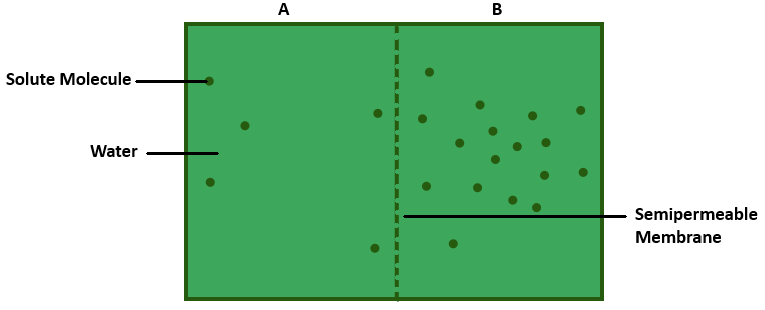If one chamber has a ${{\psi }_{w}}$ of -2000 kPa and the other -1000kPa, which is the chamber that has the higher $\psi$ ?Verified
119.1k+ views
Hint: Water potential is an actual measurement of the potential energy in water or the difference in potential energy between a given water sample and pure water (at atmospheric pressure and suitable temperature).

Water potential totally depends on the number or concentration of water molecules. More the number of water molecules in a system, higher will be the water potential. Hence, pure water has the highest water potential. By convention water potential of pure water has been fixed as zero. This means that the water potential of any solution will always be negative or less than zero. It is represented by ${{\psi }_{w}}$ , and the value of ${{\psi }_{w}}$ is measured in bars, pascals or atmosphere. Water always moves from the area of high water potential or high energy to the area of low water potential or low energy, i.e., from less negative potential (-1000kPa) to more negative potential (-2000kPa). The movement of a substance from an area of higher free energy to an area of lower free energy is called diffusion and when it occurs through a semipermeable membrane, it is called osmosis. The chamber having Pressure potential of -1000kPa has a higher water potential. A solution that has a higher water potential is called a dilute solution
So, in the above example chamber, A has higher water potential or higher $\psi$.